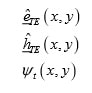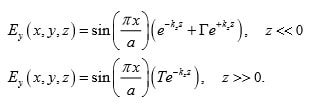# ECE 6340 Homework 5

Assignment: Please do Probs. 1-8 and 11-12 from the set below. You are welcome to do other problems for your own benefit.

Note: Assume that the permeability is that of free space in all problems, unless otherwise noted. Also, assume that the cross section of the waveguide is arbitrary unless otherwise noted. (You do not need to know the cross-sectional shape to answer the questions, unless the problem gives you this information.)

1. For each part below, specify which system (coaxial cable, waveguide, fiber-optic cable, or antenna system) that you think would be best suited for the job, and give a short explanation of why (justify your answer in each case). Keep in mind issues such as loss, distortion, power-handling capability, expense, and ease of connection.
• To carry a 1 kHz signal from a signal generator to an oscilloscope.
• To carry the VHF/UHF signal from your rooftop antenna to your TV set.
• To carry a high-speed internet signal across the entire city with minimum loss and distortion.
• To communicate with astronauts on the International Space Station.
• To carry power at 2.54 GHz from the magnetron inside your microwave oven to the oven cavity where the food is placed.
• To carry a digital signal from a computer to a printer on the same table.
• To connect a microstrip antenna to a vector network analyzer, assuming a frequency of 2.0 GHz. The VNA has coaxial output connectors.
• To carry a 60 GHz millimeter-wave signal across a room with minimum attenuation, given that we wish to connect to the waveguiding system with coaxial connectors.
• To connect several computers to a base station, assuming that all of the computers are located in different rooms of the same house, and that it is not desirable to drill holes in the walls.
• To connect directly to a horn antenna.
2. The dominant mode in an air-filled waveguide has a cutoff frequency of 2.0 GHz. Find the value of kc for this waveguide mode.
3. The waveguide as in the previous problem is now filled with lossless Teflon, having a relative permittivity of 2.2. Find the new value of kc for the same waveguide mode, and the new cutoff frequency for this mode.
4. The waveguide in the previous problem (filled with Teflon) operates at 1.5 GHz. What is the normalized phase constantβ/k0 for the waveguide mode at this frequency?
5. A certain waveguide is air filled. The attenuation of the dominant mode is measured to be 1 dB/cm at 10.0 GHz. Determine β and α for the dominant mode at 10.0 GHz when the same waveguide is filled with water having εrc = 60 - j
6. Assume that we have an air-filled rectangular waveguide with dimensions a b× (the longer dimension a is the x dimension). The origin is at the lower left corner of the waveguide cross section. Determine what the following terms are in the transverse equivalent network model, for the dominant TE10 waveguide mode:Assume that the unit vectors are chosen to be real. Assume that the ψt function has been chosen so that the power flow down the waveguide (for a given value of the voltage V and current I ) is the same as that on the corresponding transmission line in the TEN model.

7. An air-filled rectangular waveguide operating at 10 GHz has dimensions a = 2.0 cm, b = 1.0 cm. The waveguide meets an identical section of waveguide that is filled with water having an effective relative permittivity εrc = 60 - j What is the (complex) reflection coefficient? What is the percentage of incident power that is transmitted into the water-filled section of waveguide?
8. As a continuation of the previous problem, what is the distance into the water-filled waveguide for which the total time-average power flow in the z direction is down by 20 dB from the total time-average power flow at the junction?
9. A waveguide is filled with a lossy material having εc = ε′c − jε′′c . A “quasi-cutoff” frequency ωc is defined as the frequency for which β = α. Show that this cutoff frequency is given by ωc√µε′c. Recall that kc is a real number, even though the waveguide is filled with a lossy material (in fact, kc does not depend on the filling material or the frequency).
10. Show that at the quasi-cutoff frequency for a TEz mode in a lossy waveguide the real power flowing down the waveguide is equal to the imaginary power flowing down the waveguide. Show that for a TMz mode the real power is approximately equal to the negative of the imaginary power, assuming that the loss tangent of the material in the waveguide is small. (Hint: Use the relation between complex power and wave impedance, and consider what happens to the wave impedance at the quasi-cutoff frequency.)
11. A rectangular waveguide carries the TE10 mode which has a wave impedance of Z0 = 500Ω at the frequency if operation. This waveguide meets a “black box” that is centered at z = 0. It is unknown what is inside the black box. The same waveguide exits and continues from the black box. From measurements, it is known that fields inside the waveguide far away from the black box are described as follows:It is found from measurements that the reflection coefficient is Γ = -0.027027 +j(0.16216) and that the transmission coefficient is T = 1 + Γ. From this information, determine what the equivalent circuit for the black box is in the TEN model, and draw a picture of the corresponding TEN circuit showing the transmission line and the circuit representation of the black box, with all impedance values labeled.

12. An X-band rectangular waveguide has dimensions a = 2.0 cm, b = 1.0 cm. An air-filled section of the waveguide meets an identical section that is filled with lossless Teflon having a relative permittivity of 2.2. A TE10 mode at 10.7 GHz (a / λ0 = 0.714) is incident on the boundary from the air-filled section. It is desired to put an inductive post in the air-filled portion of the waveguide at a distance d from the boundary, in order to have a perfect match seen by the incident waveguide mode. Determine the distance d and also the value of the post reactance Xp (in Ω) in the TEN model that are necessary for a match. Use the smallest value of d It is recommended that you use the Smith chart to solve this problem.
13. For any waveguide, we know that a TEz mode is orthogonal to a TMz mode (orthogonal in the complex power sense, as discussed in Notes 13). Two modes of the same type (both TEz or both TEz) are also orthogonal is they are not degenerate (i.e., they are orthogonal if they have different wavenumbers). If two modes of the same type are degenerate, they may or may not be orthogonal. While the above statements are always true, we can make some further statements if we know something about a particular waveguide. For example, consider a rectangular waveguide. Two modes of the same type will be orthogonal provided they do not have the same values for both of the indices (m,n). That is, they will be orthogonal if at least one of the two indices is different between the two modes. Prove that this is true, using the concept of orthogonality of the relevant sin or cosine functions.